Courses

# Gate Mock Test- 9: CS/IT

## 65 Questions MCQ Test GATE Computer Science Engineering(CSE) 2022 Mock Test Series | Gate Mock Test- 9: CS/IT

Description
This mock test of Gate Mock Test- 9: CS/IT for GATE helps you for every GATE entrance exam. This contains 65 Multiple Choice Questions for GATE Gate Mock Test- 9: CS/IT (mcq) to study with solutions a complete question bank. The solved questions answers in this Gate Mock Test- 9: CS/IT quiz give you a good mix of easy questions and tough questions. GATE students definitely take this Gate Mock Test- 9: CS/IT exercise for a better result in the exam. You can find other Gate Mock Test- 9: CS/IT extra questions, long questions & short questions for GATE on EduRev as well by searching above.
QUESTION: 1

### Wayne Rooney …………… after netting the ball in the final seconds of the game.

Solution:

Exulted- to express great pleasure or happiness, especially at someone else's defeat or failure

QUESTION: 2

### Choose the most appropriate pair of words from the options given below to complete the following. Despite all the rhetoric about building a new democracy, and the ________ of bringing freedom and ________ to the country, the invasion has only resulted in increased violence and hardship.

Solution:

In the sentence a word that is complementary to 'freedom' is required. Hence, 'piece' which means a portion can be eliminated. This leaves us with options 2 and 3. It can be gathered from the sentence that invasion resulted in violence and hardships though it promised positive things like democracy, peace and freedom. So all these promises were false ideas. Thus, 'illusion' best fits here, making option 2 the correct answer.

An 'allusion' is an indirect or passing reference.
E.G. The Renaissance writers alluded to literature written during the Classical Greek period.

QUESTION: 3

### Continue the sequence 2, 5, 10, 17, 28, 41, _, _, _

Solution:

The relation among the given numbers is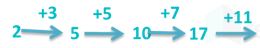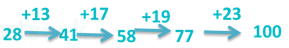Hence, the next numbers are 58, 77, 100.

*Answer can only contain numeric values
QUESTION: 4

A point on AC in the following triangle such that ∠ADB = ∠ABC. Then BD in (cm) is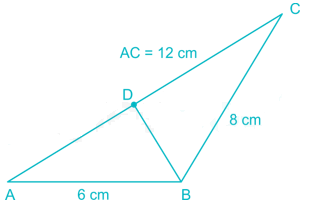Solution:

By similar triangles ΔADB and ΔABC, we get

⇒ DB/8=6/12

⇒ DB = 48/12 = 4 cm

∴ BD = 4 cm

QUESTION: 5

The numerical value of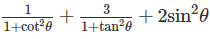will be

Solution:

Given expression is,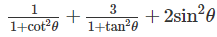Using: 1 + tan2θ = sec2θ and 1 + cot2θ = cosec2θ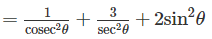Using: cosec θ = 1/sin θ and sec θ = 1/cos θ

= sin2θ + 3 cos2θ + 2 sin2θ

= 3sin2θ + 3cos2θ

= 3(sin2θ + cos2θ)

Using: sin2θ + cos2θ = 1

= 3 × 1 = 3

QUESTION: 6

An advance team of the elite special operations unit was sent to make a reconnaissance of the area before the main strike team reached the scene.

Which of the following is a statement that can be inferred from the facts stated in the above statement

Solution:

The statement provided to us in the question states the fact that an initial team was sent for the reconnaissance of the target area before the main strike team. It is to be noted here that the word ‘reconnaissance’ means observation of an area to get information about it.

Now, nowhere in the sentence is there any mention of the relative training or the equipments of the two teams, neither is there any indication to asses this fact. Therefore, it is safe to eliminate options 1 and 4.

Now, the third statement indicates that the strike team could not work without the main team. There is no evidence in the sentence to point out to any such fact. It is simply stated that the initial team was sent to make reconnaissance before the main team, in order to assist them. It cannot be assumed that the job of the initial team was absolutely essential for the second team.

The second inference states that the team did not posses sufficient and complete information about the target area. This is an inference that can be safely drawn from the given statement, since if they would have had enough information, there would have been no need for an initial team.

Hence, option 2 is the correct answer.

QUESTION: 7

Based on the distribution of surface area of the Earth at different elevations and depths (with reference to sea - level) shown in the figure, which of the following is FALSE?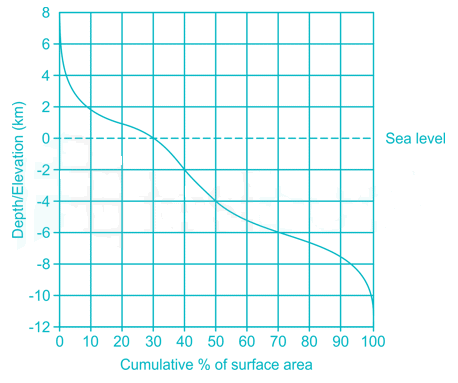Solution:

From graph it is evident that option 1 is true.

From graph, Of the surface area above sea - level, larger proportion lies below 2 km elevation

From graph, Of the surface area below sea - level, larger proportion lies below 4 km depth.

Option 4 is true as maximum depth is 12 km and maximum elevation is 8 km.

∴ Option 3 is FALSE

QUESTION: 8

Ananth takes 6 hours and Bharath takes 4 hours to read a book. Both started reading copies of the book at the same time. After how many hours is the number of pages remaining to be read by Ananth, twice that the number of pages remaining to read by Bharath? Assume Ananth and Bharath read all the pages with constant pace.

Solution:

Given, Ananth takes 6 hours and Bharath takes 4 hours to read a book.

Let the number of pages of the book be n.

∴ In 1 hour, Ananth reads n/6 pages of the book while Bharath reads n/4 pages of the book.

Let after ‘t’ hours the number of pages remaining to be read by Ananth is twice that number of pages remaining to read by Bharath.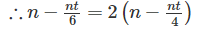⇒ 1 – t/6 = 2 – t/2

⇒ t/3 = 1

⇒ t = 3 hours

*Answer can only contain numeric values
QUESTION: 9

In a group of 11 people, 5 are wearing white shirt, 5 are wearing black shirt and one is wearing red shirt. Find the number of ways they can sit around a circular table so that no two people wearing same color shirt sit together.

Solution:

Number of ways 5 people can sit around round table = (5-1)! = 4!Now 5 spots are created for other 5 people wearing black white shirt.

∴ Total ways = 4! × 5!Now for each arrangement 10 spots are created for the person wearing Red shirt.

∴ Total ways = 4!5!10 = 28800

QUESTION: 10

Find the probability of a number which is divisible by either 5 or 3 out of first 500 natural Odd Numbers.

Solution:

The First 500 Natural Odd numbers are given as 1,3,5,7,…………….999.

The Numbers divisible by 3 from the above list are given as 3,9,15,21…………999

The above series is in Arithmetic progression,

Tn = a+(n−1)d

999 = 3+(n3−1)6 ⇒ n3 = 167

So total Numbers which are divisible by 3 in the given list is, n3 = 167

The Numbers divisible by 5 from the above list are given as 55,15,25,35…………99

The above series is in Arithmetic progression,

Tn = a+(n−1)d

995 = 5+(n5−1)10 ⇒ n5 = 100

So total Numbers which are divisible by 5 in the given list is, n3 = 100.

The Numbers divisible by 15 from the above list are given as 15,45,75,105…………975

The above series is in Arithmetic progression,

Tn = a+(n−1)d

975 = 15+(n15−1)30 ⇒ n15 = 33

So total Numbers which are divisible by 3 in the given list is, n3 = 33

So Required Probability p = p(n3)+p(n5)−p(n15) =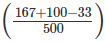= 0.468

QUESTION: 11

Consider the following proposition:

(p→q)∧(q→r)→(p→r)∨(r→p)

Above proposition is a

Solution: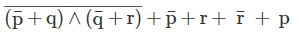≡ 1

Hence it is a tautology.

*Answer can only contain numeric values
QUESTION: 12

Given S = {a, b, c, d}.

Number of functions possible on S which are neither one-one nor onto is:

Solution:

Total number of functions = 44 = 256

Number of functions which are either one -one or onto = 4! = 24

Number of functions which are neither one -one nor onto​ = 256 - 24 = 232

QUESTION: 13

Consider the following grammar:

G = S → SS|ab|ba|aba|bab|ϵ

Which of the following string is not generated by above grammar?

Solution:

baabbabb can not be generated using above grammar.

*Answer can only contain numeric values
QUESTION: 14

The following numbers are inserted into an empty binary search tree in the given order:

10, 1, 3, 5, 11, 12, 6

What is the height of the binary search tree?

Solution:

BST has sorted in-order traversal, 10-1-3-5-6 will be the longest path from root to leaf. Hence height of BST will be 4.

QUESTION: 15

A network with CSMA/CD protocol in the MAC layer is running at 1 Gbps over a 1 km cable with no repeaters. The signal speed in the cable is 2x108 m/sec. The minimum frame size for this network should be:

Solution:

S ≥ 2 × bandwidth × td ≥ 2 × 109 × 1000/2 × 108 ≥ 10000bits

QUESTION: 16

Which of the following is an LL(1) conflict?

Solution:

FIRST/FIRST Conflict for LL(1)

S -> E | E 'a'

E -> 'b' | ε

FIRST(E) = {'b', ε} and FIRST(E 'a') = {'b', 'a'}

Thus in LL(1) table, there is conflict under terminal 'b' of production rule S.

S -> A 'a' 'b'

A -> 'a' | ε

The FIRST set of A now is {'a', ε} and the FOLLOW set {'a'}.

QUESTION: 17

Which of the following sorting algorithm will be worst choice to sort a linked list?

Solution:

To sort a linked list, Merge sort is the best choice and Heap sort is impractical.

QUESTION: 18

The set of values of p for which the roots of the equation 3x+ 2x + p(p – 1) = 0 are of opposite sign is

Solution:

p(p−1) < 0, because product of roots is a negative number.

Thus p must be less than 1 and greater than 0

QUESTION: 19

Which of the following operations is performed more efficiently by doubly linked list than by singly linked list?

Solution:

If pointer to the node to be deleted is given, delete operation is more efficient in doubly linked list O(1) than singly linked list O(n), because to delete a node in singly listed list, pointer to the previous node is needed. To get this previous node, we have to traverse the list. But in doubly linked list we can get the previous node using previous pointer.

*Answer can only contain numeric values
QUESTION: 20

Suppose that the maximum transmit window size for a TCP connection is 12000 bytes. Each packet consists of 2000 bytes. At some point of time, the connection is in slow-start phase with a current transmit window of 4000 bytes. Subsequently, the transmitter receives two acknowledgements. Assume that no packets are lost and there are no time-outs. What is the maximum possible value of the current transmit window? (in bytes).

Solution:

In slow-start phase, for each ACK, the sender increases the current transmit window by Maximum Segment Size (MSS). As we are given a packet consists of 2000 bytes and that can be taken as MSS. So, after two ACKs, current transmit window:

= 4000 + 2000 + 2000

= 8000

QUESTION: 21

Which of the following is true for an undirected graph G = (V,E) such that every vertex has degree greater than 1?

Solution:

For making sure that the graph is having every vertex degree greater than 1 have to add the edge that will repeat at least one vertex causing cycle.

QUESTION: 22

Consider set S = {The set of all rational numbers including zero} and operation

I. addition II. Multiplication III. Division

Under which operation the set will form a group?

Solution:

Multiplication will not form a group because inverse of zero does not exist.

Division operation will not form a group because n/0 is not defined.

QUESTION: 23

Consider a DRAM chip connected to a channel having 8 memory banks. A bank contain 16K rows. DRAM is refreshed once per 64ms. And refreshing operation takes 60ns(nano second). How many refresh operation performed in 1 sec?( 1 sec ≈ 1.024 sec).

Solution:

Total number of rows in the bank is 214. Total number of banks is 8. And in 1.024 sec total number of refresh operation performed by the controller is 1024/64 =16.

Total number of refresh operation performed by the controller is

214 × 8 × 16 = 221

*Answer can only contain numeric values
QUESTION: 24

The minimum number of bits required to represent - 64 in 2’s complement representation is _________.

Solution:

Whenever a number is in 2n form then the minimal 2’s complement representation is 1 followed by n number of zeros.

So, – 64 = – 26 = 1000000

QUESTION: 25

What is the broadcast address of the first sub network in a class-C network assigned with an IP address - 207.35.7.0 (The subnet mask is 255.255.255.248)

Solution:

negate subnet mask and perform logical or operation with given IP address.

QUESTION: 26

The following C function takes a simply-linked list as input argument.

typedef struct node {

int value;

struct node *next;

}

node *p;

int a;

return 0;

while(p→next!= NULL){

p=p→next;

if(p→value < a)

a = p→value;

}

return a;

Which of the following is true for the above function?

Solution:

The function returns minimum value from the list

QUESTION: 27

Which of the following languages is/are context-free?

I. {anbmcndm│n,m≥0}

II. {anbnbmam│n,m≥0}

III. {anbmcn│n,m≥0}

Solution:

I is context sensitive language

II and III are CFLs.

QUESTION: 28

Which of the following regular expression describes the language

L = {w is in (1 + 0)* │ w ends with 10}?

I. (1*0*)*10

II. (0 + 1(1 + 01)*00)*1(1 + 01)*0

III. (1*0*)*100*

Solution:

III can not generate L rest can.

QUESTION: 29

Consider the following program:

a = 1;

b = 2;

c = 3;

c = a + b;

b = a + c;

d = b + c;

e = d + a;

Assuming that all operations take their operands from registers, what is the minimum number of registers needed to execute this program without spilling?

Solution:

To avoid spilling we have to use 3 register

a = 1;

b = 2;

c = 3;

c = a + b;  r1 = c, r1 = b, r2 = a

b = a + c r2 = a, r1 = b, r3 = c, because we will using the value a,b,c in future

d = b + c;

e = d + a;

return d + b;

QUESTION: 30

In a data link layer, bit stuffing is used in transferring data. If the sent data after bit stuffing is 001111101101011111001111 and the flag is 01111110, then what will be the data after destuffing?

Solution:

As the flag is 01111110, we have to delete 0 after every five consecutive 1’s.

*Answer can only contain numeric values
QUESTION: 31

A channel has 10 Kbps bit rate using stop and wait protocol and has propagation delay of 4 ms. For a frame with 400 bit, what will be the efficiency (in percentage)?

Solution:

transmission time for 400 bits = 40ms

utilization =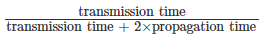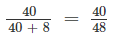= 83%

QUESTION: 32

Which of the following set is empty?

Solution:

There does not exist any function f(n) such that f(n) ϵ o(g(n)) and f(n)ϵ ω(g(n)) at the same time.

Both the sets in option(A) are mutually exclusive.

QUESTION: 33

foo (int n, int A[ ])

{

int i = 0, j = 0

for (i = 0, i ≤ n, i ++)

while (j<n && A[i] < A[j])

j++;

}

Time complexity of the function foo () is_____

Solution:

‘j’ is initialized just once in the given program. Also, the loop is iterated for 'n' times making the time complexity as O(n).

QUESTION: 34

Give the breadth first traversal of the given graph below starting from vertex A.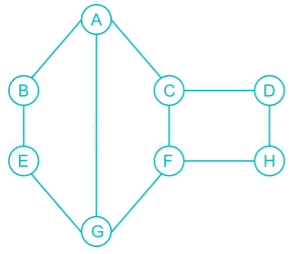Solution:

The algorithm for BFS traversal of a graph is like:

First the starting vertex us enqueued. Then, the following steps are repeated until the queue is empty:

1. Remove the vertex at the head of the queue and call it ‘vertex’.

2. Visit ‘vertex’

3. Follow each edge emanating from ‘vertex’ to find the adjacent vertex and call it ‘to’. If  ‘to’  has not already been put into the queue, enqueue it.

According to the above algorithm, the BFS traversal of the above graph.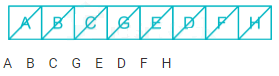*Answer can only contain numeric values
QUESTION: 35

Sum of eigen values of matrix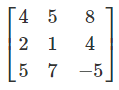is ________

Solution:

Sum of eigen values of a matrix = trace of the matrix

As trace of the given matrix is = 4 + 1 - 5 = 0.

Hence sum of the eigen values = 0.

QUESTION: 36

The essential prime implicates of F(A,B,C,D) = ∑m(0,1,5,7,10,14,15) are

Solution:

F(A,B,C,D) = ∑m(0,1,5,7,10,14,15)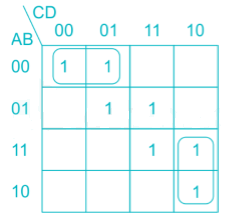To cover 0, 10 min terms,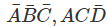are essential.

*Answer can only contain numeric values
QUESTION: 37

The modulus of Following asynchronous counter is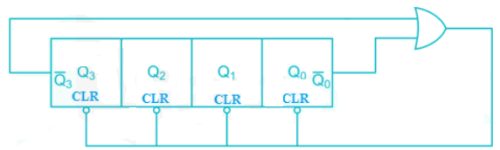Solution:

For QQQQ0 = 1 0 0 1

The output of OR gate = 0 hence all Flip – Flops are cleared

So the counting sequence is as follows

0000, 0001,0010 …… 1000, 0000, 0001…………..

∴ Modulus of counter = 9

QUESTION: 38

Power of a is computed using following method:

an = a.an-1 when n is odd

an = (an/2)2 when n is even

Number of multiplications required to compute a28 is:

Solution:

a2 = a ∗ a

a3 = a ∗ a2

a6 = a3 ∗ a3

a7 = a ∗ a6

a14 = a7 ∗ a7

a28 = a14 ∗ a14

QUESTION: 39

Consider the following code which is used to detect the loop in the linked list. Find the missing statement A?

while(A)

{

if(p == q) exit(0) //loop detected

p = p->next;

q = (q->next)?(q->next->next) : q->next;

}

Solution:

q will go end of list first and will accept

q = NULL and will terminate.

Otherwise p and q both get a valid pointer value and keep moving.

QUESTION: 40

Consider the following propositions:

(i) (~p → ~q) → (q → p)

(ii) [p ∧ (p∧~q∨~r∧s ∨ q∧r∧s ∨p) →~r] → (r→~p)

(iii) (p → q) ∧ (~p → ~q) → (p ↔ q)

Which of the above proposition/s is/are a tautology?

Solution:

(i) Tautology: Because contrapositive of an implication always follows from it.

(ii) Tautology: LHS is nothing but p→~r which in turn implies RHS.

(iii) Tautology: (p → q) ∧ (q → p) ≡ (p ↔ q)

*Answer can only contain numeric values
QUESTION: 41

On a TCP connection, current congestion window size is 4 KB. The window size advertised by the receiver is 6 KB. The last byte sent by the sender is LastByteSent = 10240 and the last byte acknowledged by the receiver is LastByteAcked = 8192. The current window size at the sender is___(in bytes)

Solution:

As Receiver window size is 6KB and network congestion window size is 4KB so sender has to send only 4KB window.

Sender window size = min(Window_congestion,Window_adverstised) = min(4KB,6KB) = 4KB

Go through this guide.

*Answer can only contain numeric values
QUESTION: 42

The following key values are inserted into a B+  tree in which order of the internal nodes is 3, and that of the leaf nodes is 2, in the sequence given below. The order of internal nodes is the maximum number of tree pointers in each node, and the order of leaf nodes is the maximum number of data items that can be stored in it. The B+  tree is initially empty.

10, 3, 6, 8, 4, 2, 1

The maximum number of times leaf nodes would get split up as a result of these insertions is___(Assume right biasing).

Solution:

Using right biasing there will be three splits:

first after inserting 6

second after inserting 8

third after inserting 2.

QUESTION: 43

The following C function takes a single-linked list of integers as a parameter and rearranges the elements of the list. The function is called with the list containing the integers 1, 2, 3, 4, 5, 6, 7 in the given order. What will be the contents of the list after function completes execution?

struct node {

int value;

struct node *next;

};

void rearrange(struct node *list) {

struct node *p, *q;

int temp;

if (!list || !list -> next) return;

p = list; q = list -> next;

while(q) {

temp = p -> value; p->value =  q -> value;

q->value = temp; p = q ->next;

q = p? p ->next : 0;

}

}

Solution:

The loop is interchanging the adjacent elements of the list.

But because of (p = q -> next;) after each interchange, next interchange starts from the unchanged elements.

1st iteration 1 2 3 4 5 6 7  ------>  2 1 3 4 5 6 7

2nd iteration 2 1 4 3 5 6 7

3rd iteration 2 1 4 3 6 5 7

p will point to 7, and q=p true, hence q = p->next = null.

QUESTION: 44

#include<stdio.h>

void function(int num){

if(num>0) {

function(--num);

printf("%d",num);

function(--num);

}

}

int main() {

function(3);

return 0;

}

Output of the above function is:

Solution:

The recursion tree for the given program is given below: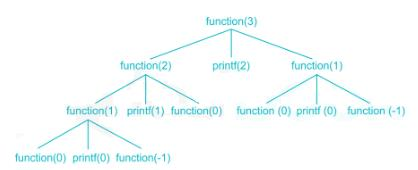Expanding the recursion carefully and moving from top to down and left to write, we can see that it will print 0120.

*Answer can only contain numeric values
QUESTION: 45

int fun(int num)

{

int result =0;

if(num <= 1)

return 1;

else

{

for(i=num;i>=1;i--)

result+=fun(i/3);

}

return result;

}

Value returned by fun(6) is:

Solution:

f(0)=1

f(1)=1

f(2)=2

i=6 result=2

i=5 result=3

i=4 result=4

i=3 result=5

i=2 result=6

i=1 result=7

QUESTION: 46

In a box of red and blue balls 2% of red and 3% of blue balls are broken. There are 30% blue balls in the box. If a ball is selected and is broken, the probability that the ball is blue is:

Solution:

E1 = Ball is red

E2 = Ball is blue

A = Ball is broken

Given P (E1) = 70/100 = 0.7, P (E2) = 30/100 = 0.3

P (A/E1) = 2/100 = 0.02, P(A/E2) = 3/100 = 0.03

By Baye’s theorem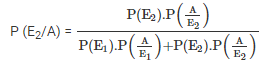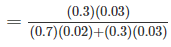= 0.39≅0.4

*Answer can only contain numeric values
QUESTION: 47

Consider a 32 bit processor which is implemented with 16 bit external data bus and driven by 50MHz input clock. (Assume processor has a bus cycle whose minimum duration is 4 input clock cycles). Then the maximum data transfer rate this processor can accomplish is___________(in MBps)

Solution:

Clock cycle =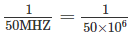= 20ns

Bus cycle = 4 × 20 = 80ns

2 Bytes are transferred (16 – bit data) for every 80 ns.

Thus the transfer rate =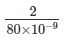= 25MBps

QUESTION: 48

Let us consider four processes P1, P2, P3, and P4. There are three resources available R1, R2 and R3 with the following number of instances

R1:9

R2:3

R3:6

The MAX_REQD matrix: the matrix showing the maximum number of resources required by each of the processes to finish is given below: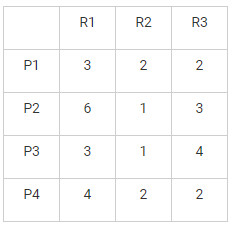The CUR_HOLDING matrix: the matrix showing the resources currently held by the processes.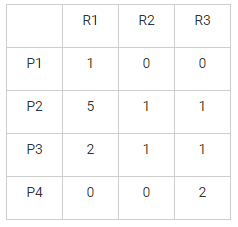What is the safe sequence using Banker’s algorithm?

Solution: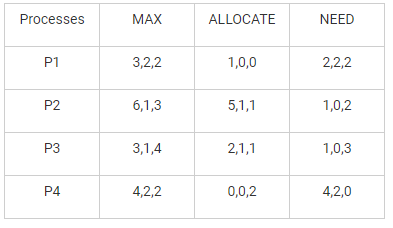number of available resources: 1, 1, 2

So, safe sequence is <P2, P3, P4, P1>

QUESTION: 49

Consider a relation with seven attributes ABCDEGH. The following dependencies are given .

AB -> C, AC -> B, AD -> E, B -> D, BC -> A, E -> G.

What is the key ?

Solution:

AC -> B           So, (AC)+ = {A,C, B,}

B -> D               (AC)+ = {A,C, B, D}

AD -> E             (AC)+ = {A,C, B, D, E}

E -> G                (AC)+ = {A,C, B, D, E, G}

So to get H we have to include H into (AC)

So the key is ACH.

QUESTION: 50

Consider a synchronous instruction pipeline with 4 stages (S1, S2, S3 & S4 ). The delay of each stages is 1.2ns, 1.3ns, 1.5ns and 2ns. The pipeline also has buffer placed between the stages. The delay for the buffer is fixed for all and requires 1ns. How much time is required to execute 10000 instructions?

Solution:

Since the pipeline is synchronous each stage will take equal delay and that delay will be 2ns (The stage taking longer time)

Since the buffer delay is 1 the first instruction will come out in 12ns

2 + 1 + 2 + 1 + 2 + 1 + 2 + 1 = 12ns

Rest 9999 instruction will be completed in every 3ns that is 9999´ 3ns = 29997ns

QUESTION: 51

Which one of the following is a key factor for preferring B-trees to binary search trees for indexing database relations?

Solution:

A disk block contains a fairly large number of keys. Unlike BST where each node contains only one key, B-Tree is designed to contain a large number of keys so that tree height is small.

QUESTION: 52

All page frames are initially empty, and a process is allowed three page frames in memory, and references its pages in the order 1,2,3,2,4,5,2,3,2,2,4,1,5.

If the page replacement policy is optimal replacement policy, then page faults caused by this process is,

Solution:

Optimal replacement policy looks forward in time to see which frame to replace on a page fault. Thus it is not a real replacement algorithm. It gives us a reference of number of frame for a given static frame access sequence.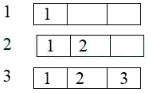2     HIT (page number 2 already in memory so there is no page faults.)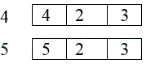2     HIT

3     HIT

2     HIT

2     HIT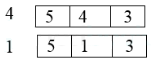5     HIT

So there are 7 page faults

*Answer can only contain numeric values
QUESTION: 53

A Btree index is to be built on the studID attributes of the relation student assume that all StudID are of 10 bytes and pointer is of 5 bytes and block size is of 512 byte. Given this scenario, then the best choice of the degree of Btree is _______.

Solution:

For Btree.

5 x + 10(x – 1) < 512

5x + 10x – 10 < 512

15x < 522

x = 34 (approx)

QUESTION: 54

Consider the following cooperating process using semaphore S and T.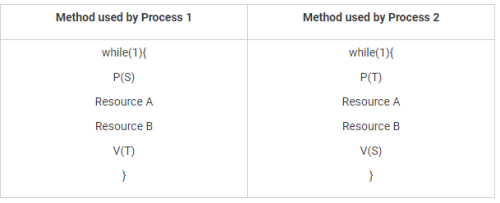Which of the following is true?

Solution:

for S = 1 and T = 0 P1 will first use the resource A, B

QUESTION: 55

Consider a memory is consists of 1024 frames (0 to 1023), each frame contains  64B and byte addressable. An array of size A is stored in the memory. A is at frame 1 byte 0. What is the address of A?

Solution:

Since the first element A   is at frame 1 and byte 0

Before reaching A  the array element A element has been stored

So total 60 complete row already been stored containing 60 ´ 512 + 100 = 30820 elements

Number of blocks required is 30820/64 = 481.5625.c

So we required total 481 complete block so address will go on 482nd block.

*Answer can only contain numeric values
QUESTION: 56

Consider a pipelined system with four stages: IF, ID, EX, WB.

Following chart shows the clock cycles required by instruction to complete each stage.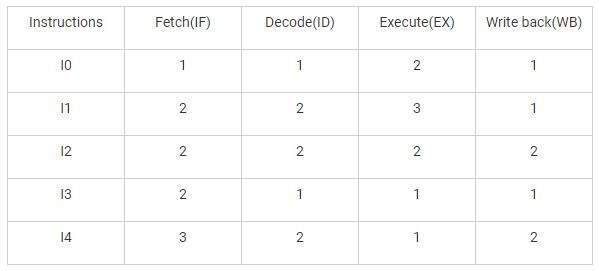How many clock cycles are required to complete the instructions?

Solution: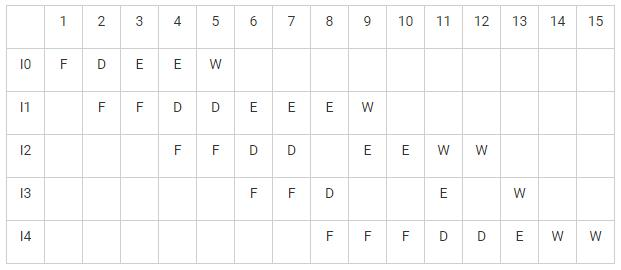It requires 15 clock cycles.

QUESTION: 57

Apply Dijkstra’s algorithm for the following graph: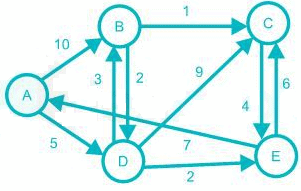Initially the set S contains the vertex A, i.e. S = {A}.

Finally, in which of the following order the vertices will be included in S, if array P holds the shortest distance from source to each vertex, then what will be P[A - E]?

Solution:

Source vertex is A. From A smallest distance is D and it is 5

The next smallest is from D to E, so the total distance from A to E is 7

The next smallest from D to B, so the total distance from A to B is 8

The next smallest from B to C, so the total distance from A to C is 9

QUESTION: 58

Consider the schema:

Flight(Flight_no, from, to, dist, depart, arrive)

Aircraft(aid, aname, cruising_range)

Certified(pid, aid)

Pilot(pid, pname, salary)

Select P.pname

From Aircraft A, Certified C, Pilot P

Where A.aid = C.aid and P.pid = C.pid and A.cruising_range > 3000

and P.pid not in (Select C2.pid

From Certified C2, Aircraft A2

Where C2.aid = A2.aid and A2.aname = ‘Boeing’)

The above query returns

Solution:

P.pid not in (Select C2.pid

From Certified C2, Aircraft A2

Where C2.aid = A2.aid and A2.aname = ‘Boeing’)

The above subquery returns the pid of those pilots who are not certified on any Boeing.

Select P.pname

From From Aircraft A, Certified C, Pilot P

Where A.aid = C.aid and P.pid = C.pid and A.cruising_range > 3000

the above subquery returns the name of the pilots who can operate with a range greater than 3000 Km.

*Answer can only contain numeric values
QUESTION: 59

Assume X is a random variable representing the output of a fair dice.

Also

Y = max (2, min (5, X))

What is the variance of Y ?

Solution: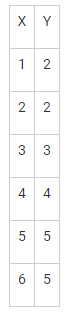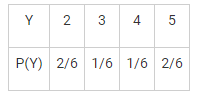E(Y) =Σ Y.P(Y) = 3.5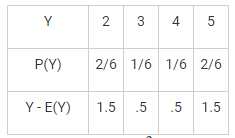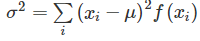= (1.5)2(2/6) + (0.5)2(1/6) + (0.5)2(1/6) + (1.5)2(2/6) = 1.583

QUESTION: 60

Consider a UNIX-like file system implemented with i-nodes that resides on a disk of size 512 GB. Each i-node has a total of 15 block addresses consisting of direct and indirect block addresses.

Suppose the implementation wants to support file size up to 1 GB using only direct block addresses and single indirect block address. At least how many of the 15 block addresses should be used as single indirect block address.

Solution:

Each i-node has a total 15 block addresses which means 15 bit block addresses.
So each i-node size = (2^15)B.

total no. of i-node = Total Size / I-node Size

= 512 GB/ (2^15)B

= (2^39)/(2^15)

= 2^24

i.e. 24’ bits are required to address the entire disk space -> 3 bytes
Block Entry Size = 3 Bytes

the number of i-node or blocks required for 1 GB file

= File Size/ I-node Size

= 1 GB/ (2^15)B

= (2^30)/(2^15)

=2^15

size required to address 2^15 blocks = No_of_blocks * Block_Entry_Size = (2^15)*3 Bytes

size required to address 1 block:

= (2^15)*3 / (2^15) = 3 block addresses

QUESTION: 61

Consider the below grammar

S →  AaB

A →  ab | a

B →  b

The above grammar is

Solution: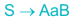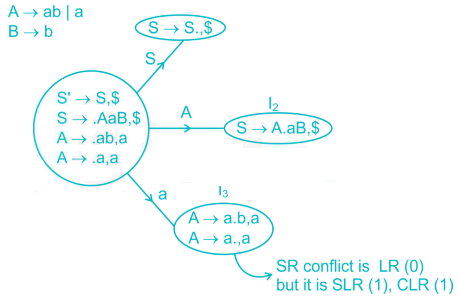It is not LR(0), but SLR(1), CLR(1).

QUESTION: 62

Match the following :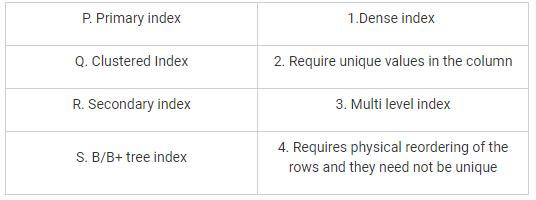Solution:

Self-explanation.

QUESTION: 63

A cache aware sorting algorithm sorts an array of size 2k with each key of size 4 Bytes. The size of the cache memory is 128 Bytes and algorithm is the combination of merge sort and insertion sort to exploit the locality of reference for the cache memory(i.e. will use insertion sort when problem size equals cache memory).

Worst case running time of the algorithm is:

Solution:

It will use merge sort at upper level and when size of the subarray will reach the size of the cache memory if will start to use Insertion sort,

∵ cache can hold 32 keys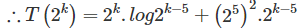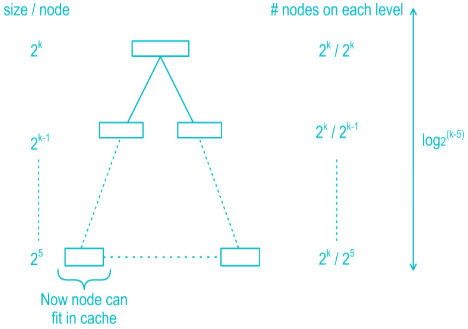∴ Merge sort in worst case will take time = height × number of elements in the array

= log2(k-5)× 2k

After that insertion sort will take (25)for each node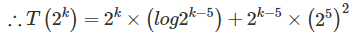QUESTION: 64

Consider the languages L1={0m1m0n|m,n>0}, L2={0m1n0n|m,n>0} and L = L1 ∪ L2 over the alphabet {0,1}. Which of the following statements is/are true?

I. L is unambiguous.

II. L is inherently ambiguous.

III. L can be recognized by a PDA..

IV. L can be recognized by a DPDA.

Solution:

L1 and L2 are context free languages. Hence, L is also context free, recognized by some

push-down automaton, because CFL's are closed under Union.

The subset {0n1n0n|n⟩0} of L is the set of strings that has two distinct derivations:

one coming from L1, and the other coming from L2. It turns out that every grammar

that describes L will remain ambiguous for at least some strings of the form 0n0n0n

Hence, L is inherently ambiguous.

*Answer can only contain numeric values
QUESTION: 65

Determine the size of the ROM of required to implement the following –

F(A,B,C) = ∑m(1,2,4,7)

F2(A,B,C) =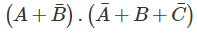Solution: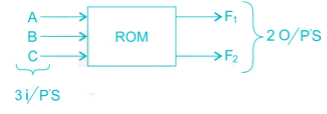3i/p,s

⇒ Size of ROM =2X×y

Where x = no. of inputs

y = no. of outputs

⇒ Size = 23 × 2 = 16